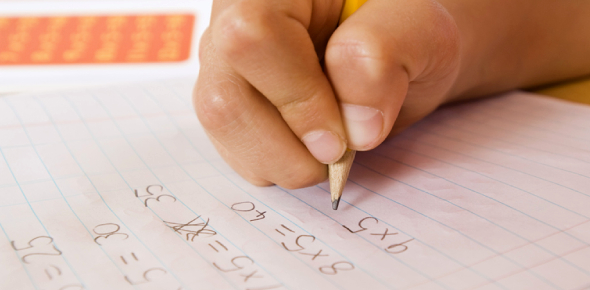# Mathematics MCQ Test Quiz!

20 Questions | Total Attempts: 424Settings.

• 1.
Find The HCF of 9, 15, and 24.
• A.

2

• B.

6

• C.

3

• D.

7

• 2.
Write in words 1,347,000.
• A.

One million four hundred and forty-seven thousand

• B.

One million three hundred and forty-seven thousand

• C.

one million seven hundred and thirty-four thousand

• D.

One thousand three and forty-seven

• 3.
Find the LCM of 20, 24, and 30.
• A.

120

• B.

40

• C.

60

• D.

74

• 4.
Find the sum of 695 and 455 and then divide the result by the difference between 39 and 34.
• A.

245

• B.

230

• C.

259

• D.

247

• 5.
Multiply 13/5 and 13/18.
• A.

13/14

• B.

14/17

• C.

17/45

• D.

45/52

• 6.
The 5th multiple of 6 is ……
• A.

36

• B.

18

• C.

30

• D.

24

• 7.
Find the sum of 29.34 and 837.5.
• A.

1020.9

• B.

867.84

• C.

1030.9

• D.

866.84

• 8.
Express  84 as a product of prime factors.
• A.

2 x 2 x 7

• B.

2 x 2 x 3 x 7

• C.

2 x 3 x 3 x 7

• D.

2 x 3 x 7

• 9.
• A.

456

• B.

465

• C.

564

• D.

546

• 10.
Which one of these numbers is not a common factor of 12 and 24?
• A.

5

• B.

2

• C.

4

• D.

3

• 11.
Change to decimal 3/8.
• A.

0.573

• B.

0.753

• C.

0.375

• D.

0.537

• 12.
Evaluate 3 2/5 + 2 2/3.
• A.

151/15

• B.

516/15

• C.

55/16

• D.

61/15

• 13.
What is the value of 1 ½ X 2 1/3
• A.

4 1/3

• B.

3 ½

• C.

5 1/3

• D.

4 ½

• 14.
Find the common factors of 15 and 20.
• A.

1, 5

• B.

1, 3

• C.

1, 2

• D.

1, 4

• 15.
10/3 is a fraction called …..
• A.

Proper fraction

• B.

Improper fraction

• C.

Equivalent fraction

• D.

Mixed fraction

• 16.
MCM in Roman numeral stands for ……
• A.

2100

• B.

2000

• C.

1800

• D.

1900

• 17.
Add the following 1.23 + 10.23 + 100.23.
• A.

3.2553

• B.

111.69

• C.

325.53

• D.

11.169

• 18.
Change to mixed fraction  14/9
• A.

14/9

• B.

15/9

• C.

91/5

• D.

51/9

• 19.
Write as decimal 4/8   (A) 0.357  (B) 0.377  (C) 0.375  (D) 0.5
• A.

0.357

• B.

0.377

• C.

0.375

• D.

0.5

• 20.
Express 5/7 as an equivalent fraction.
• A.

10/14

• B.

6/7

• C.

7/14

• D.

4/7

Related TopicsBack to top